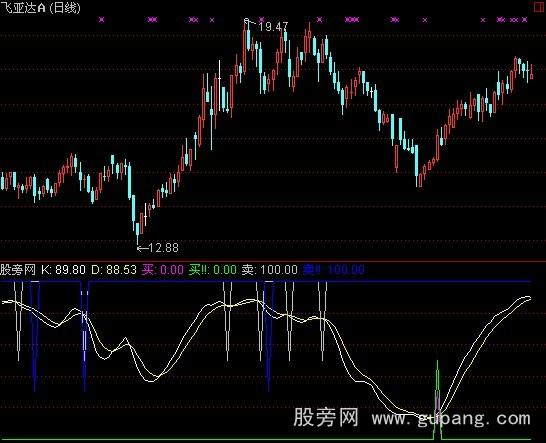# 通达信傻子炒股指标公式

N:=27;M1:=3;M2:=3;M3:=3;

VAR1:=(CLOSE-LLV(LOW,N))/(HHV(HIGH,N)-LLV(LOW,N))*100;

VAR3:=SMA(VAR1,M1,1);

K:SMA(VAR3,M2,1);

D:SMA(K,M3,1);## 相关阅读

《通达信傻子炒股指标公式》由网友“昔瞳”推荐。